# 音频D类功放LC滤波器设计（一）

TI公司也有介绍D类放大器LC滤波器的设计文档，文末会分享出来。我写的与TI的区别，TI的主要介绍具体如何设计，我主要想说明思路过程，并指出里面的一些细节，为什么是这样。我希望的是，有了思路，即使没有任何文档，遇到类似的问题，也能自己去分析。

D类功放工作原理

D类功放相对于A，B，C类来说更不好理解，因为它是需要调制的，看起来就是占空比不同的PWM波，波形看着与我们的音频模拟波形一点都不像。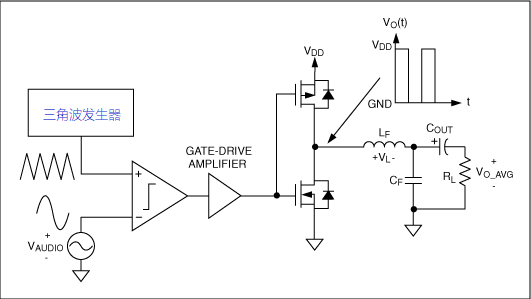LC滤波器该如何设计，L，C如何取值？

D类放大器，我们常用的方式是差分的方式，即两个MOS对管中间接喇叭。下面就只分析这种差分方式，单端的分析方法也差不多。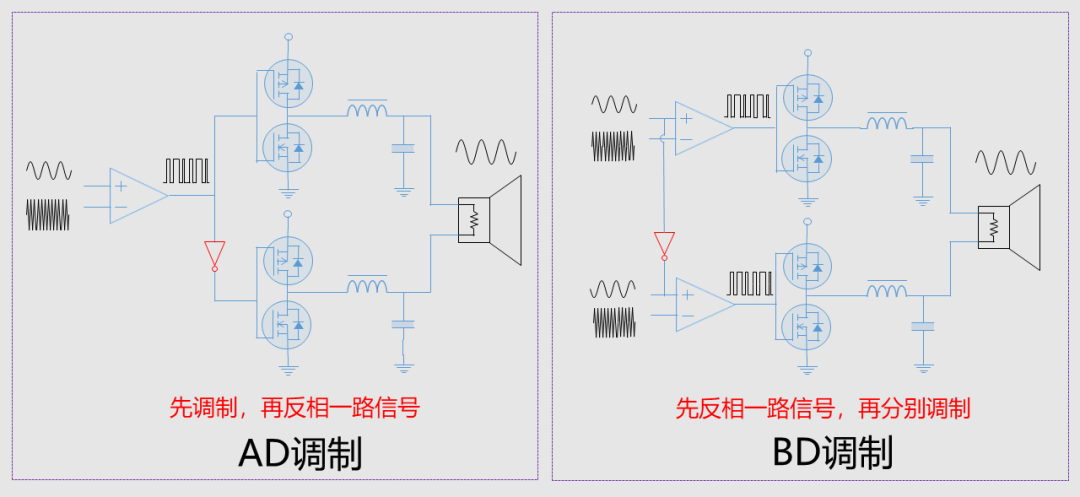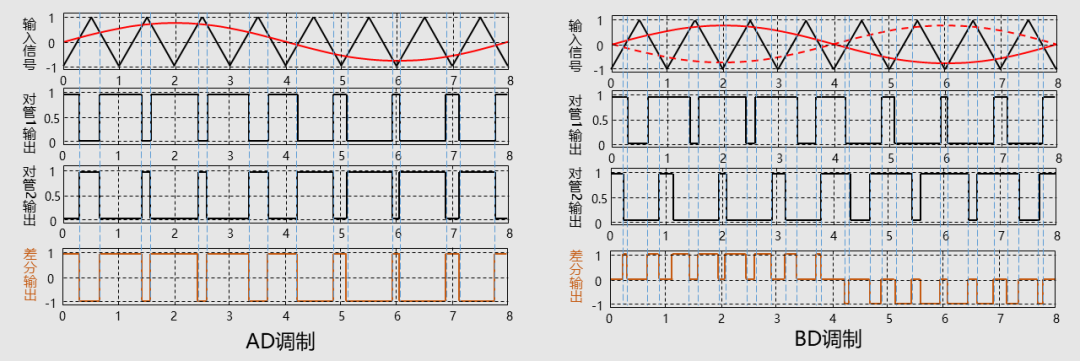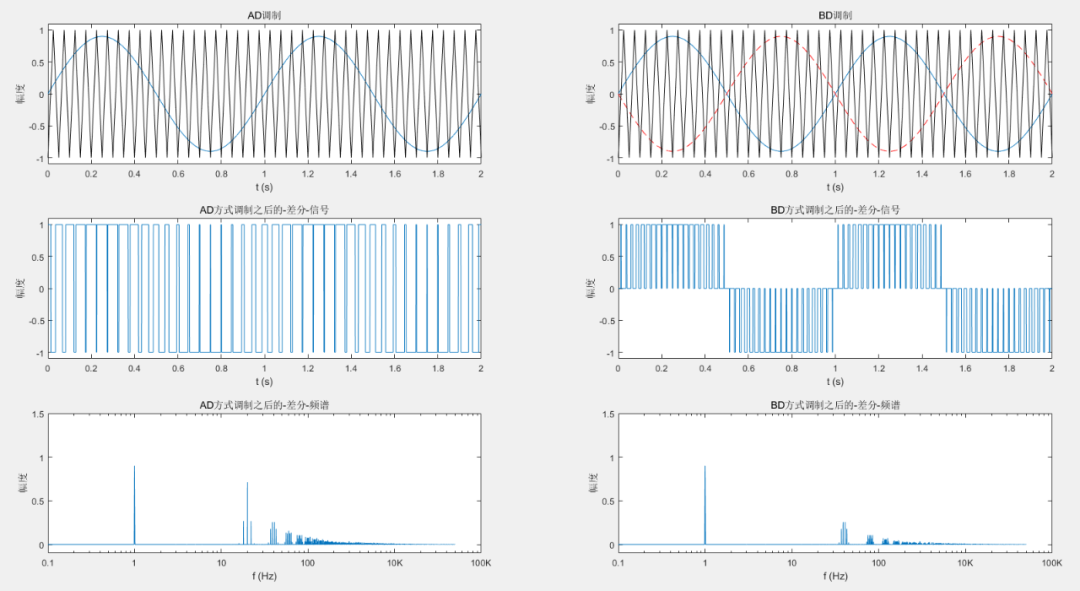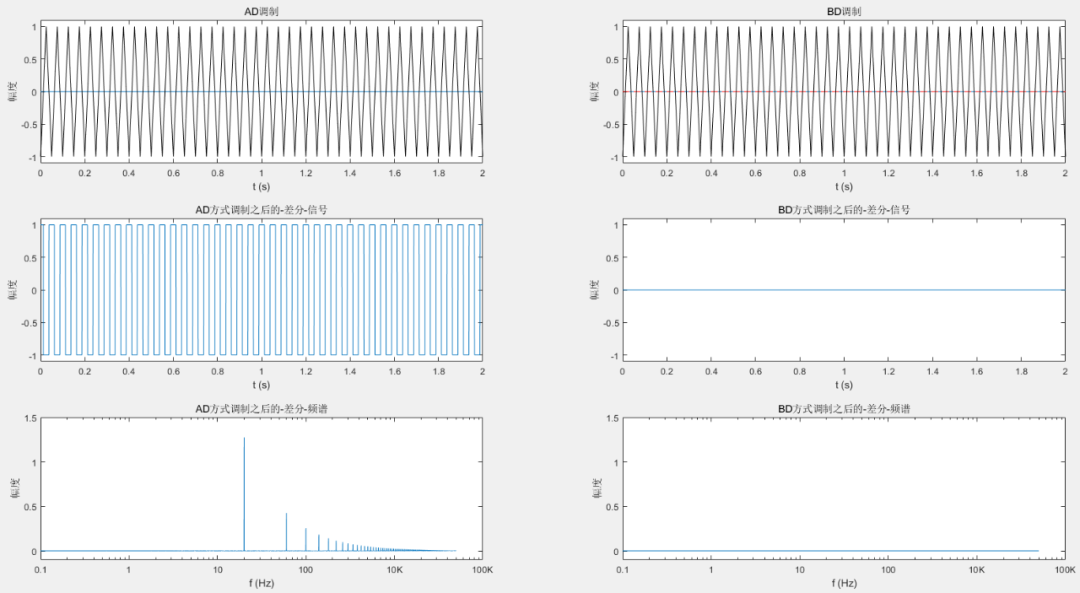``````f_audio=1;      %被调制信号（音频信号）频率为1Hz
f_sanjiao=20;   %三角波调制频率为20Hz

%%%%%%%%%%%%%%%%%%%%%%%%  fft采样设置
Fs=10000;  %采样率为Fs
L=(Fs/f_audio)*100;   %信号长度(采样总点数)：100个周期的信号，长度越长，fft精度越高，但是执行时间越长
T=1/Fs;    %采样周期
t=(0:L)*T; %时间长度

A_audio = 0.9;   %音频信号的幅度为 0.9----可以修改为不同的值尝试
S1=A_audio*sin(2*pi*f_audio*t);         %被调制信号(音频信号)为幅度A_audio的正弦波
S2=sawtooth(2*pi*f_sanjiao*t,0.5);  %调制信号（三角波）为幅度为1的三角波

N=length(t);
PWM2=zeros(1,N); %定义PWM2的长度  BD调制后差分波形
tmp=zeros(1,N);  %定义tmp的长度  计算用（中间变量）
for i=1:N
if S1(i)>S2(i)
PWM1(i) = 1;
tmp(i)  = 1;
else
PWM1(i) = -1;
tmp(i)  = 0;
end
end

for i=1:N
if -S1(i)>S2(i)
PWM2(i) = tmp(i)-1;
else
PWM2(i) = tmp(i);
end
end

subplot(3,2,1);
plot(t,S1,t,S2,'k');
set(gca,'XLim',[0 2/f_audio]);%x轴的数据显示基频2个周期
set(gca,'YLim',[-1.1 1.1]);
xlabel('t (s)');
ylabel('幅度');

subplot(3,2,3);
plot(t,PWM1);
set(gca,'XLim',[0 2/f_audio]);%x轴的数据显示范围
set(gca,'YLim',[-1.1 1.1]);
xlabel('t (s)');
ylabel('幅度');

X1=abs(fft(PWM1));
subplot(3,2,5);
semilogx(Fs*(0:(L/2))/L,X1(1:L/2+1)*2/L);
set(gca,'XLim',[0.1 10000]);%x轴的数据显示范围
set(gca, 'XTickLabel' ,{'0.1','1','10','100','10K','100K'}); %x轴频率数据
set(gca,'YLim',[-0.1 1.5]);
xlabel('f (Hz)');
ylabel('幅度');

%%%%%%%%%%%%%%%%% BD调制差分信号
subplot(3,2,2);
plot(t,S1,t,-S1,'--r',t,S2,'k');
set(gca,'XLim',[0 2/f_audio]);%x轴的数据显示基频2个周期
set(gca,'YLim',[-1.1 1.1]);
title('BD调制');
xlabel('t (s)');
ylabel('幅度');

subplot(3,2,4);
plot(t,PWM2);
set(gca,'XLim',[0 2/f_audio]);%x轴的数据显示范围
set(gca,'YLim',[-1.1 1.1]);
title('BD方式调制之后的-差分-信号');
xlabel('t (s)');
ylabel('幅度');

X2=abs(fft(PWM2));
subplot(3,2,6);
semilogx(Fs*(0:(L/2))/L,X2(1:L/2+1)*2/L);
set(gca,'XLim',[0.1 10000]);     %x轴的数据显示范围
title('BD方式调制之后的-差分-频谱');
set(gca, 'XTickLabel' ,{'0.1','1','10','100','10K','100K'}); %x轴频率数据
set(gca,'YLim',[-0.1 1.5]);
xlabel('f (Hz)');
ylabel('幅度');``````

0/200